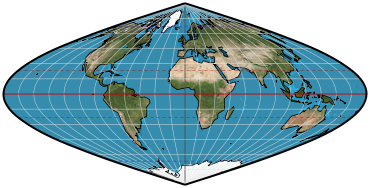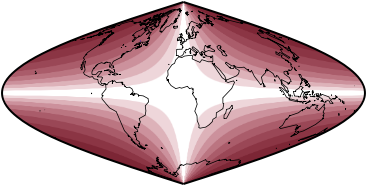## Directory of Map Projections

What is a projection?

Previous | Next

equal-area polynomialParameters: Note, Coefficient a, Coefficient b, Coefficient c, Coefficient d, Coefficient e

Classifications

pseudocylindric
equal-area

Graticule

Meridians: Complex curves equally spaced along parallels.
Parallels: Straight parallel lines.
Poles: Points.
Symmetry: Symmetric east-west. Symmetric north-south when b and d = 0.

Limiting forms

Sinsoidal, when a = 1.0 and the others = 0.

Parameters

Coefficients a, b, c, d, e, each for a successively higher power of the latitude. Generally these values should be 1.0 or less, and the higher the letter, the smaller the value.

Origin

Developed by Daniel “daan” Strebe (1962–) in 2015. However, the concept is straightforward, with several polynomial developments appearing in the literature, typically for optimizing the projection in some way.